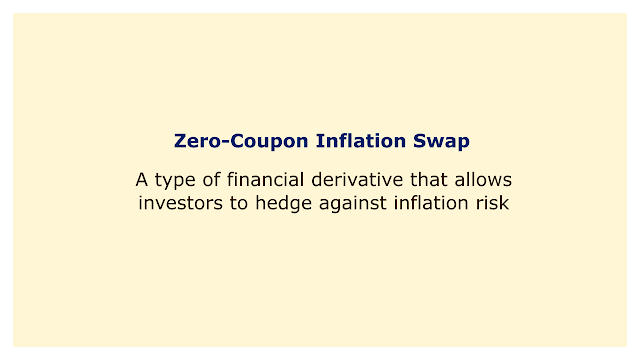# Zero-Coupon Inflation Swap (ZCIS)Image: Moneybestpal.com

### A zero-coupon inflation swap (ZCIS) is a type of financial derivative that allows investors to hedge against inflation risk. A ZCIS is an agreement between two parties to swap a fixed payment for a variable payment dependent on the inflation rate at the end of a predetermined period. A ZCIS only requires one payment at maturity as opposed to normal inflation swaps, which require recurring payments.

#### How does a ZCIS work?

There are two legs on a ZCIS: a fixed leg and an inflating leg. The notional amount and contract fixed rate establish how much the fixed leg will pay, which is a predetermined sum. The inflation leg pays a sum that changes according to the CPI's change during the course of the contract. A basket of goods and services average price level is measured by the CPI.

The payer of the fixed leg (or seller) consents to pay the fixed sum to the buyer (or payment of the inflation leg) at maturity. The payer of the inflation leg consents to pay the fixed leg payer the variable sum at maturity. The discrepancy between the variable amount and fixed amount is the net cash flow at maturity.

The formula for calculating the fixed amount is:

Fixed amount = Notional amount x [(1 + Contract fixed rate)^Contract period - 1]

The formula for calculating the variable amount is:

Variable amount = Notional amount x [CPI at maturity / CPI at start - 1]

As an illustration, let's say that two parties sign a ZCIS with a notional sum of \$100 million, a contract fixed rate of 2%, and a contract duration of 5 years. The CPI is 100 at the outset of the contract and 110 at maturity. The fixed amount is:

Fixed amount = \$100 million x [(1 + 0.02)^5 - 1] = \$10.4 million

The variable amount is:

Variable amount = \$100 million x [110 / 100 - 1] = \$10 million

The net cash flow at maturity is:

Net cash flow = Fixed amount - Variable amount = \$10.4 million - \$10 million = \$0.4 million

The fixed leg payer pays \$0.4 million to the inflation leg payer.

#### Why use a ZCIS?

A ZCIS can be used as a hedge against inflation risk or as a way to make predictions about expected inflation in the future. For instance, a buyer of a ZCIS who anticipates higher inflation may be entitled to a higher payment should inflation increase over the set rate specified in the contract. On the other hand, if an investor anticipates a decline in inflation, they can sell a ZCIS and make a reduced payout if inflation falls below the fixed rate of the contract.

Other inflation-linked instruments, including inflation-linked bonds or options, can also be priced using a ZCIS. A ZCIS's contract fixed rate represents the market's forecast for inflation over the contract's duration. For pricing other items, this rate might be utilized as a discount factor or an inferred forward rate.
Tags